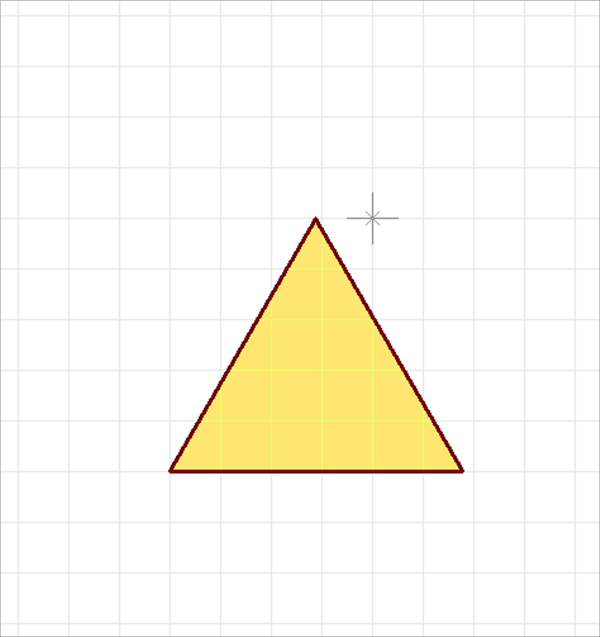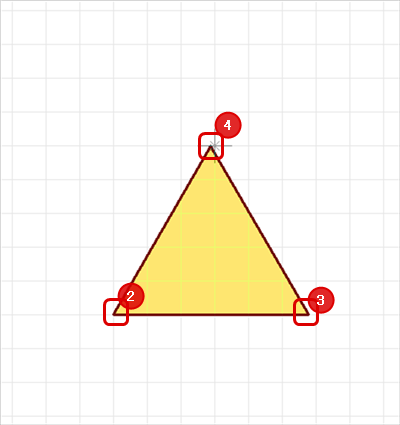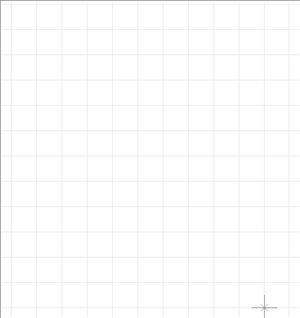## Schematic Capture : Draw

### Drawing Equilateral Triangles

With Equilateral Triangle, an equilateral triangle is created by specifying the base and top.The following will explain each operation for drawing an equilateral triangle.

#### Selecting the Equilateral Triangle Menu

Select [Draw] => [Equilateral Triangle]

There are several other ways to perform this. Refer to About Executing Menus.

#### Drawing an Equilateral Triangle

After selecting the Equilateral Triangle Menu,

STEP 1: Click the base line Start Point (Base Start Point)
STEP 2: Click the base line End Point (Base End Point)
STEP 3: Click the height

 Drawing an Equilateral Triangle
 The following is the operation for an equilateral triangle.
 (1) Click [Draw] => [Equilateral Triangle]. => It will move to Equilateral Triangle mode.(2) Click the base line Start Point (Base Start Point). (3) Click the base line End Point (Base End Point). (4) Click the height.The equilateral triangle is created.#### Pushback Equilateral Triangle

When creating an equilateral triangle, you can return the corner to the previous status.

 Before Pushback After PushbackThe following is the operation for executing pushback.

Right click, Select => [Pushback]
* Press "Back" on the keyboard.

#### Editing the Line Width

When creating an equilateral triangle, you can edit the line width.

 Line Width 0.2 mm Line Width 1mmThe following is the operation for editing the line width.

STEP 1: Right click, Select => [Edit Line Width] => The "Line Width Settings" window will open.
* This can also be done by pressing "W" on the keyboard.
STEP 2: Input a Line Width, and then click "OK"

It is applied to the whole equilateral triangle before it is determined.

#### Editing Properties (Color, Line Style, etc.)

It is possible to edit the Line Width, Line Style, Line Color, and Fill Style, etc.
The following is the operation for editing.

STEP 1: Edit values in the Property Window

It is applied to the whole equilateral triangle before it is determined.

#### Canceling an Equilateral Triangle

When creating an equilateral triangle, it is possible to return to the status before drawing by canceling the equilateral triangle being created.

 Before Canceling After CancelingThe following is the operation for executing pushback.

Right click, Select => [Cancel]
* Press "Escape" on the keyboard

#### Exiting Equilateral Triangle Mode

The following is the operation for exiting Equilateral Triangle mode.

When not creating an equilateral triangle,

Right click, Select => [Cancel]
* Press "Escape" on the keyboard